# How to Calculate and Solve for the Volume of a Constituent, Volume of a Mixture and Volume Concentration in Chemistry | The Calculator Encyclopedia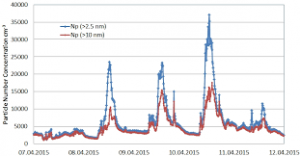The image above represents the volume concentration.

To compute the volume concentration of a substance, two essential parameters are needed and these parameters are volume of the constituent (Vi) and volume of the mixture (V).

The formula for calculating volume concentration:

φ = ViV

Where;
φ = Volume concentration
Vi = Volume of the constituent
V = Volume of the mixture

Let’s solve an example;
Find the volume concentration with a volume of the constituent of 23 and a volume of the mixture of 32.

This implies that;
Vi = Volume of the constituent = 23
V = Volume of the mixture = 32

φ = ViV
φ = 2332
φ = 0.718

Therefore, the volume concentration is 0.718.

Calculating the Volume of the Constituent when the Volume Concentration and the Volume of the Mixture is Given.

Vi = Vφ

Where;
φ = Volume concentration
V = Volume of the mixture

Let’s solve an example;
Find the volume of the constituent with a volume concentration of 18 and a volume of the mixture of 22.

This implies that;
φ = Volume concentration = 18
V = Volume of the mixture = 22

Vi = Vφ
Vi = 18 x 22
Vi = 396

Therefore, the volume of the constituent is 396 cm3.

Calculating the Volume of the Mixture when the Volume Concentration and the Volume of the Constituent is Given.

V = φ / Vi

Where;
φ = Volume concentration
V = Volume of the constituent

Let’s solve an example;
Find the volume of the mixture with a volume concentration of 24 and a volume of the constituent of 6.

This implies that;
φ = Volume concentration = 24
V = Volume of the constituent = 6

V = φ / Vi
V = 24 / 6
V = 4

Therefore, the volume of the mixture is 6 cm3.

Nickzom Calculator – The Calculator Encyclopedia is capable of calculating the volume concentration.

To get the answer and workings of the volume concentration using the Nickzom Calculator – The Calculator Encyclopedia. First, you need to obtain the app.

You can get this app via any of these means:

To get access to the professional version via web, you need to register and subscribe for NGN 1,500 per annum to have utter access to all functionalities.
You can also try the demo version via https://www.nickzom.org/calculator

Once, you have obtained the calculator encyclopedia app, proceed to the Calculator Map, then click on Basic Chemistry under Chemistry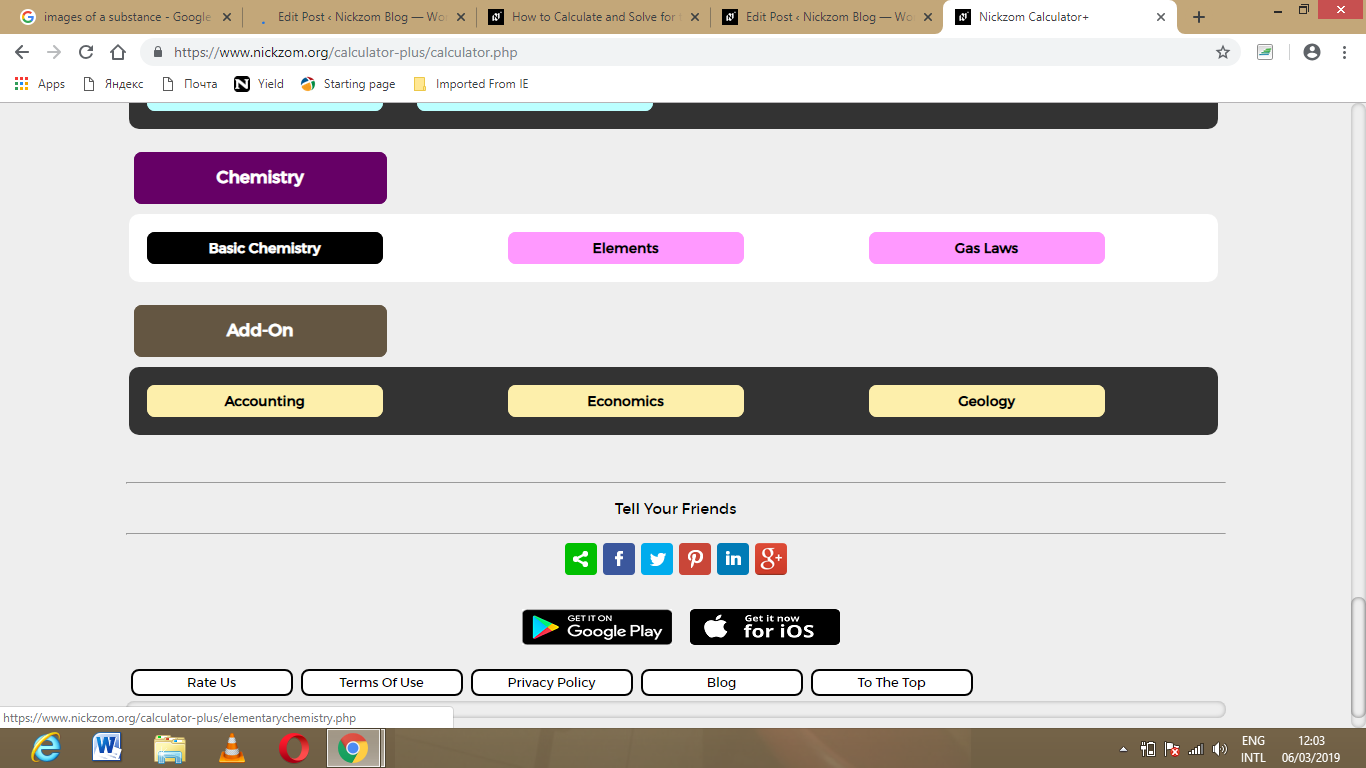Now, Click on Volume Concentration under Basic Chemistry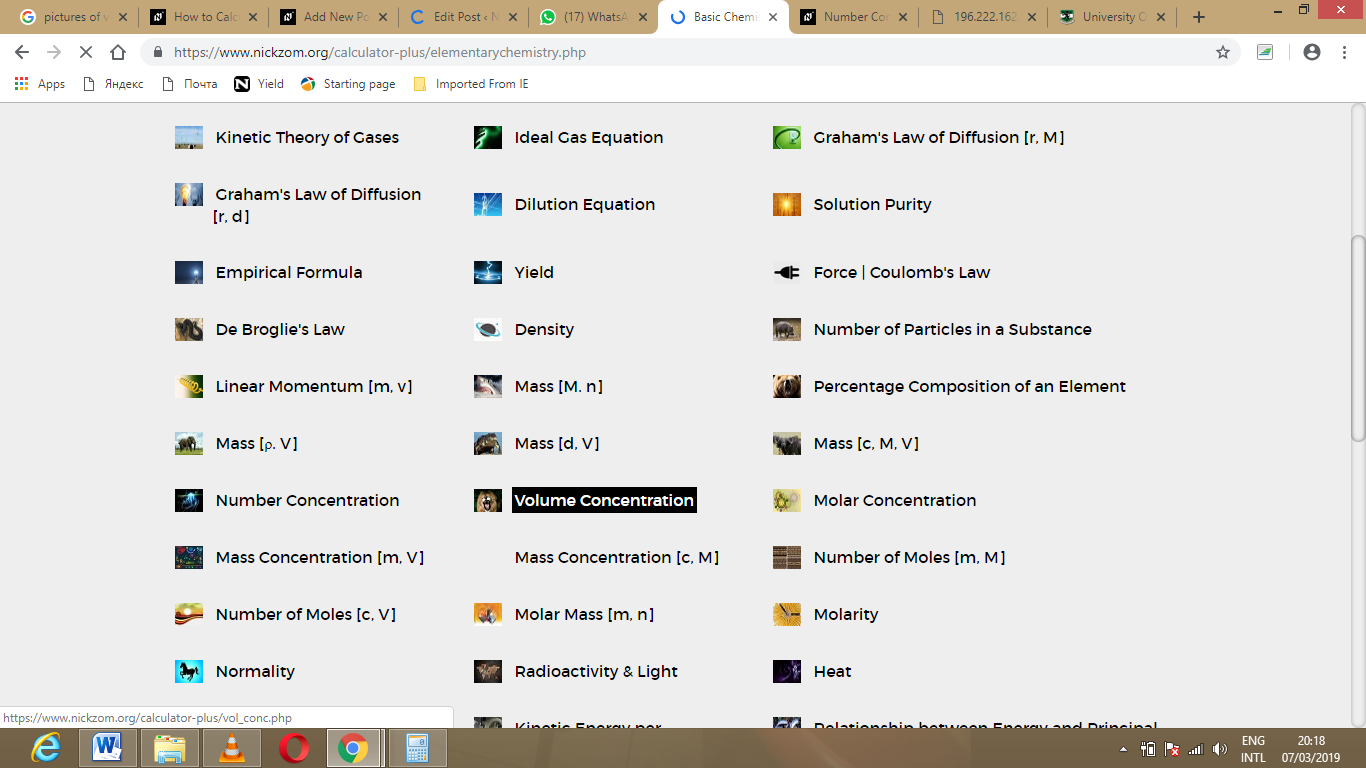The screenshot below displays the page or activity to enter your values, to get the answer for the volume concentration according to the respective parameters which are the volume of the constituent (Vi) and volume of the mixture (V).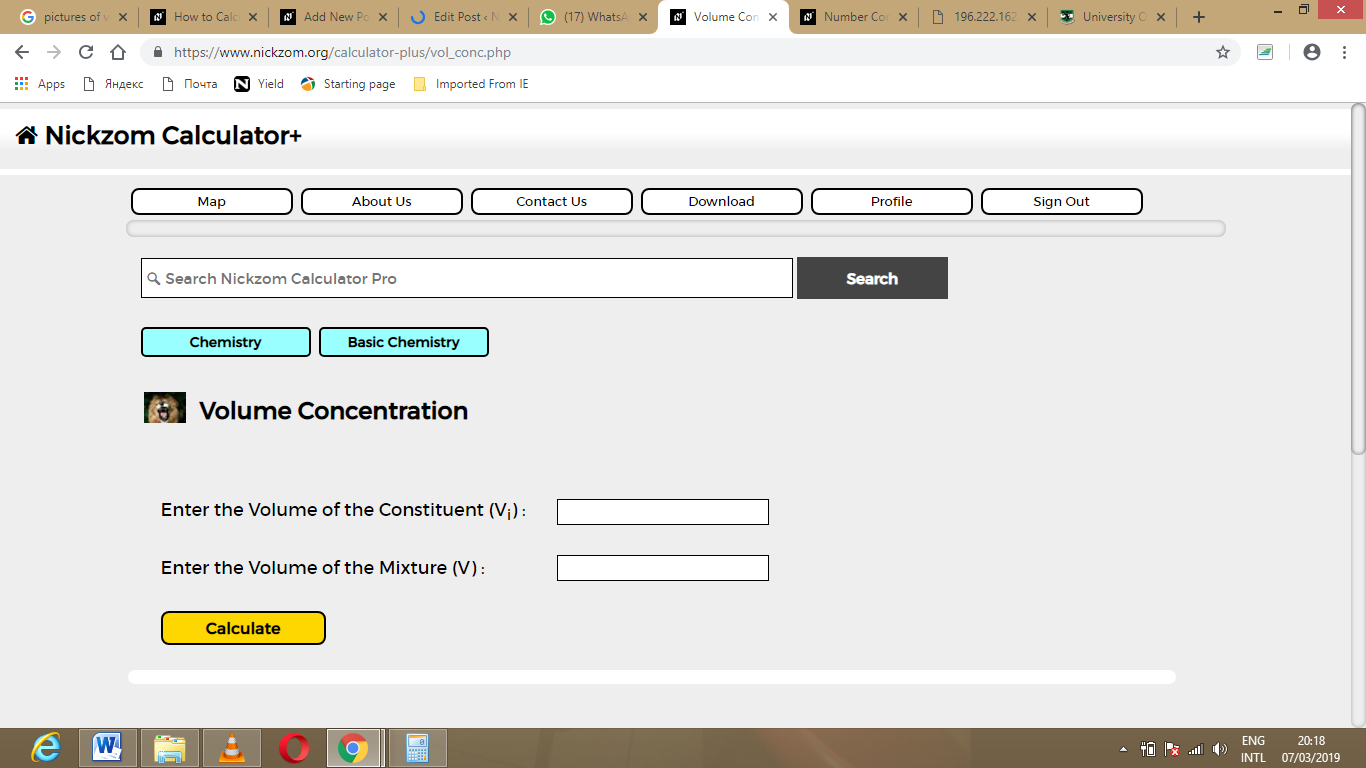Now, enter the values appropriately and accordingly for the parameters as required by the example above where the volume of the constituent (Viis 23 and volume of the mixture (V) is 32.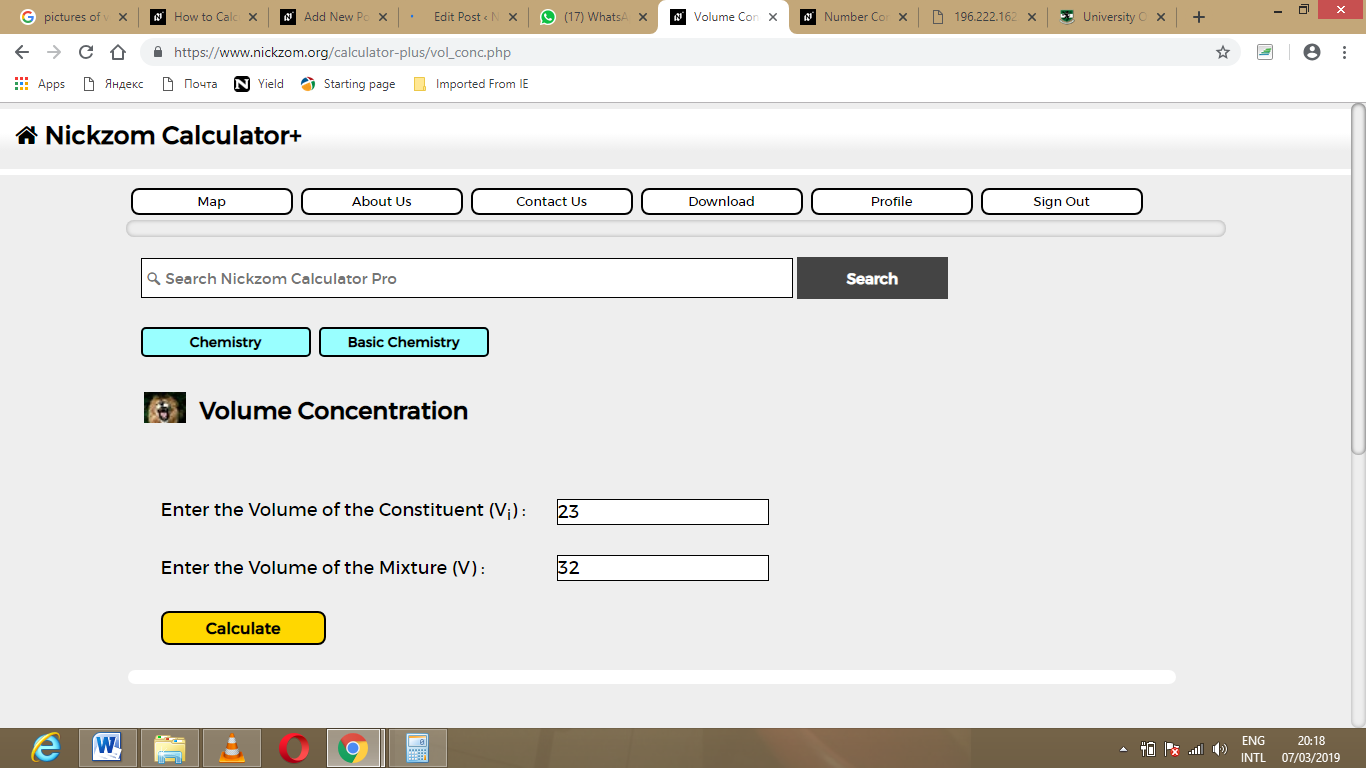Finally, Click on Calculate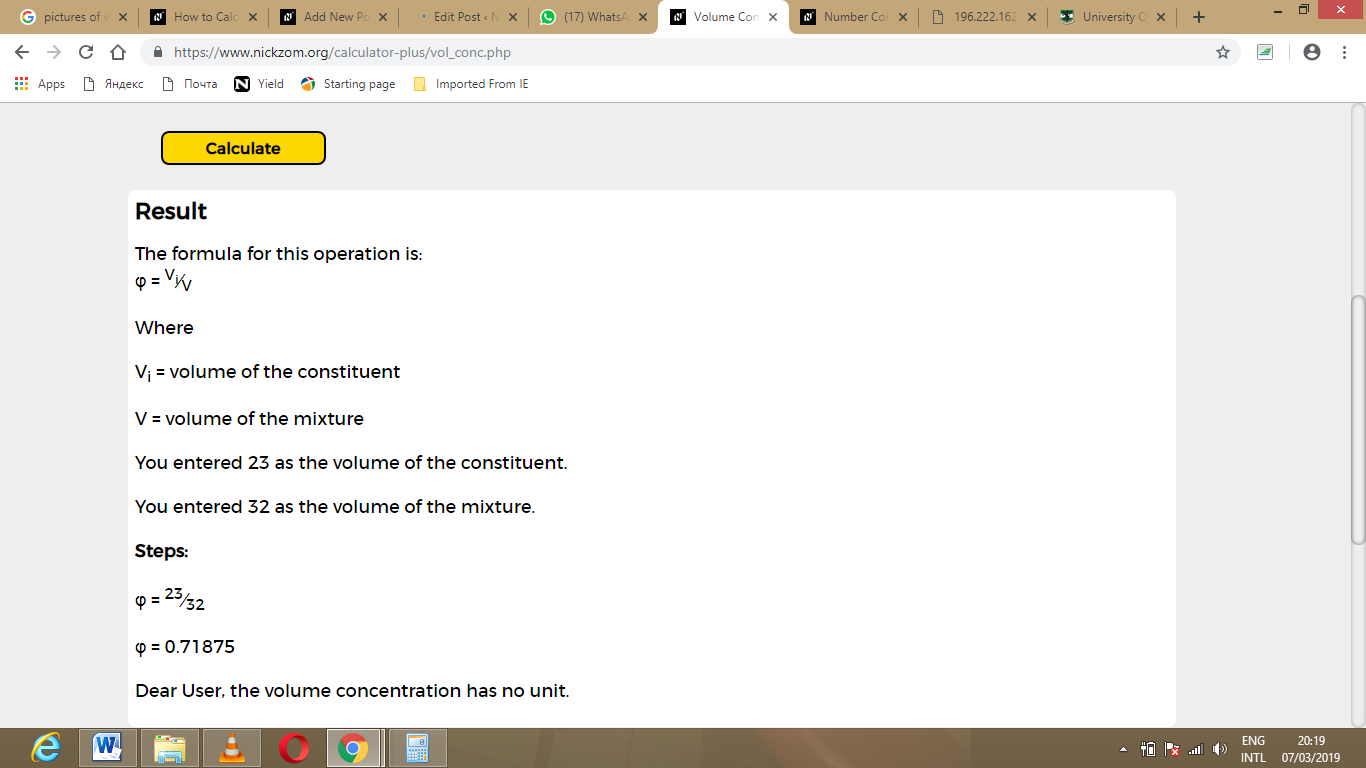As you can see from the screenshot above, Nickzom Calculator – The Calculator Encyclopedia solves for the volume concentration and presents the formula, workings and steps too.